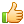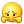A-A+

# MBA联考决胜系列之一(实数模块)

2009年08月31日 阅读 33,522 次

MBA联考决胜系列之一(实数模块)## 56 条留言  访客：56 条  博主：0 条

1.Angelina

老师很多题解析很笼统，没有具体的解释啊！！

2.ailin1068

陈老师，第14题转化成三角形公式的地方不是很明白，怎么看出与三角形面积公式相同的？

3.fmapskx

为什么叫竞赛专题？有比赛机制，还是这些题比较难？

•chenjian

就是强化提高的内容，适合想得高分的同学。

4.kangjuan2000

今天是第一次来论坛，收获不小。支持一下。5.mirandayuandq

第十六题 答案应该是705中间的那个零吧~
因为从100开始第605个数字是704 即605 100-1
要刨除100这个数字

所以第2004位是704的4
而第2006位即705的0

6.mirandayuandq

第十六题 答案应该是705中间的那个零吧~
因为从100开始第605个数字是704 即605+100-1
要刨除100这个数字

所以第2004位是704的4
而第2006位即705的0

7.cntoworld

第一个题 就看不懂啊  为什么是得出 两个平方根都等于 13 ？？

•pandashuner

cntoworld  你好。我认为此题可能书上有一点错误。这两个平方根应该互为相反数，即a^2+b^2=-(4a-6b+13),然后移项配方可得（a+2）^2+(b-3)^2=0,从而得到a=-2，b=3.代入a^2+b^2=13，这个数是13^2=169

•pandashuner

cntoworld  你好。我认为此题可能书上有一点错误。这两个平方根应该互为相反数，即a^2 b^2=-(4a-6b 13),然后移项配方可得（a 2）^2 (b-3)^2=0,从而得到a=-2，b=3.代入a^2 b^2=13，这个数是13^2=169

8.sherry_hang请教各位 很抱歉我算出来的结果和答案怎么也对不上。用平方差公式 是否是X^2-Y^2=(X Y)(X-Y).可是这样带入算出来的答案好怪。是我的理解错了吗？谢谢

9.sherry_hang请教各位 很抱歉我算出来的结果和答案怎么也对不上。用平方差公式 是否是X^2-Y^2=(X+Y)(X-Y).可是这样带入算出来的答案好怪。是我的理解错了吗？谢谢

10.q

谢谢陈老师

11.crice

第十四题我知道解法，和大家说说
因为xyz(x y z)=1，所以x y z=1/xyz
所以x y=1/xyz-z,而y z=1/xyz-x

所以(x y)(y z)=(1/xyz-z)*(1/xyz-x)=(1/xyz)平方-1/yz-1/xy xz)=(x y z)*(1/xyz)-1/yz-1/xy xz=(x y z-x-z/xyz) xz=1/xz xz
有因为x,y,z都是正数，所以1/XZ XZ大等于2根号1，那就是答案2咯

12.crice

第十四题我知道解法，和大家说说
因为xyz(x+y+z)=1，所以x+y+z=1/xyz
所以x+y=1/xyz-z,而y+z=1/xyz-x

所以(x+y)(y+z)=(1/xyz-z)*(1/xyz-x)=(1/xyz)平方-1/yz-1/xy+xz)=(x+y+z)*(1/xyz)-1/yz-1/xy+xz=(x+y+z-x-z/xyz)+xz=1/xz+xz
有因为x,y,z都是正数，所以1/XZ+XZ大等于2根号1，那就是答案2咯

13.felala

求教：
第14题比较困惑，能有更详细的解释吗？

14.麽麽喳喳

16题，我怎么算都是705的零是2006位数，而不是解答中的706，您看，100到699 占了600个三位数，然后700是601个，以此类推，704对应着605个三位数，然后再加上2个，就是705的那个零，这样对吗？

15.云淡风轻

老师，辛苦了!!!

16.yhtak

求助：
第十题：
在0-100的自然数中，含有奇数个正整数因子的自然数个数是几个？ 答案是9个。
我的困惑：例如1的正整数因子只有1一个。4=1*2*2 含有正整数因子3个。9=1*3*3 含有正整数因子3个。那么6=1*2*3 也应该是含有正整数因子3个。为什么答案给出的9个里面没有这个数呢？还是我对于答案的理解有错误。麻烦指正。谢谢！

第十四题x,y,z为正数，xyz(x y z)=1，那么(x y)(y z)的最小值是多少？
答案是当3个数相等时，取到最小值2。
我的困惑：
1，为什么3个数相等时候，表达式有最小值 。
2，当3个数相等时候，3×4=1,推出 4×2=4/3倍根号3。也不是2阿？
请指教！xiexie